Mathematical and Physical Journal
for High Schools
Issued by the MATFUND Foundation
 Already signed up? New to KöMaL?

# KöMaL Problems in Physics, January 2020

Show/hide problems of signs:## Problems with sign 'M'

Deadline expired on February 10, 2020.

M. 392. Measure the specific heat capacity of the material of a 100-forint (or 1-euro) coin in a calorimeter (thermos flask).

(6 pont)

statistics## Problems with sign 'G'

Deadline expired on February 10, 2020.

G. 693. Two exactly alike trains move towards each other (not necessarily at the same speed) along parallel railroad tracks next to each other. The length of each locomotive is the same as the length of each railway carriage. Both trains consist of 19 carriages and locomotives which haul the trains (one locomotive in front of each train). Peggy is travelling in the third carriage (counted from the locomotive). After the two trains meet, 36 seconds elapse when Peggy's carriage gets exactly next to that carriage of the other train in which Daniel is travelling, and then 44 seconds elapse until the two trains go past each other. Counted from the locomotive, in which carriage is Daniel?

(4 pont)

solution (in Hungarian), statistics

G. 694. An exactly 100-kg rocket, moving in space, ejects 100 g exhaust gas in each second. The exhaust leaves the nozzle at a speed of 1 km/s. What is the acceleration of the rocket?

(3 pont)

solution (in Hungarian), statistics

G. 695. Toy penguins were suspended to an unsteady room decoration. The very light rods (see the figure) were suspended at their quadrisection points such that the structure is in equilibrium. What are the masses of the second, the third, and the fourth toy penguins, if the first one has a mass of 480 g?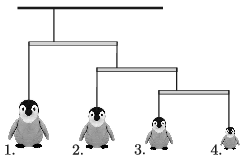(3 pont)

solution (in Hungarian), statistics

G. 696. Alexander got a soldering iron as a Christmas present, and he immediately tried it. He soldered two resistors of resistances $\displaystyle 2000~\Omega$ and $\displaystyle 500~\Omega$ in parallel, and then with this he soldered another $\displaystyle 500~\Omega$ resistor in series. Finally with this he soldered a $\displaystyle 600~\Omega$ resistor in parallel. He connected the circuit to a battery and measured the voltage across the $\displaystyle 2000~\Omega$ resistor, which was 2 V.

$\displaystyle a)$ Draw a schematic figure of the circuit.

$\displaystyle b)$ What is the voltage across the battery?

$\displaystyle c)$ What is the current through the battery?

(3 pont)

solution (in Hungarian), statistics## Problems with sign 'P'

Deadline expired on February 10, 2020.

P. 5186. The total mass of a sleigh and a child on it is 25 kg. The coefficient of kinetic friction between the sleigh and the snow is 0.05.

$\displaystyle a)$ We would like to pull the sleigh horizontally at a constant speed. What is the horizontal force that must be applied?

$\displaystyle b)$ The sleigh is accelerated from rest for 2 seconds with a constant force of 50 N along the horizontal, snow covered ground, and then it is released. What is the distance covered by the sleigh from the starting position until it again comes to rest?

(3 pont)

solution (in Hungarian), statistics

P. 5187. Two simple pendulums are fixed at the same height. They swing in parallel planes close to each other. One of them is $\displaystyle \ell_1=25$ cm, whilst the other is $\displaystyle \ell_2=1.2$ m long. The two pendulums are displaced in the opposite direction at the same small angle, and then released.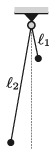$\displaystyle a)$ How long does it take for the pendulums to go past each other after they were released?

$\displaystyle b)$ How much time elapses until they meet again?

$\displaystyle c)$ What should the ratio of the lengths of the pendulums be in order that the fifth encounter be the first one when the direction of the velocities of the two pendulum bobs is the same?

(5 pont)

solution (in Hungarian), statistics

P. 5188. A cuboid-shaped prism is sliding along a horizontal surface in a straight line and is decelerating due to friction. The figure shows the velocity-time graph of its motion.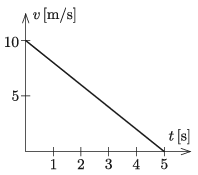A spring-loaded toy cannon is attached to the top of the prism, which fires projectiles of mass $\displaystyle m$ at a speed of $\displaystyle v_0$, during the slowing motion of the prism. The total mass of the prism and the toy cannon is $\displaystyle M$.

$\displaystyle a)$ To which direction should the cannon be aimed in order that the projection not affect the decelerating motion of the prism, or in other words the graph of the motion of the cannon continue in the same way after firing the projectile?

$\displaystyle b)$ If the angle between the gun barrel and the horizontal is adjusted to half of the angle determined in the previous problem, then how does this affect the graph of the deceleration? Sketch the modified velocity-time graph of the motion, if the projectile was shot at $\displaystyle t=2$ s.

Data: $\displaystyle m=0.1$ kg, $\displaystyle M=1$ kg, $\displaystyle v_0=5$ m/s.

(5 pont)

solution (in Hungarian), statistics

P. 5189. There is a small air bubble at the middle of a long cylinder-shaped tube, filled with glycerine. When the tube is held vertically, the air bubble is raising at a constant speed of 1 cm/s. Where will the air bubble stop in the tube when it is held horizontally and is accelerated along its symmetry axis to a final speed of 20 m/s? Where will the bubble move if the tube's speed is uniformly increased to 30 m/s, and where will it stop if the tube is ceased to move? (The short time intervals when the bubble starts and stops, and the retarding effect of the wall of the tube are negligible.)

(5 pont)

solution (in Hungarian), statistics

P. 5190. The bottom end of a vertical, thin-walled glass tube has a shape of a hemisphere. The diameter of the tube is 10 cm. The tube is filled with water up to a height of 20 cm. 30 cm above the water level and along the axis of the tube there is a small light source.

$\displaystyle a)$ Where should we place a screen in order to gain a sharp image of the light source?

$\displaystyle b)$ What is the magnification?

(The refractive index of water is $\displaystyle n= 4/3$.)

(5 pont)

solution (in Hungarian), statistics

P. 5191. Two samples of ideal gas, of the same amount, are taken through the cyclic processes shown in the figure. The figure shows the $\displaystyle p$\,–\,$\displaystyle V$ diagram of processes $\displaystyle 1\rightarrow2\rightarrow3\rightarrow1$, and $\displaystyle 1\rightarrow3\rightarrow4\rightarrow1$. If the working substance of two engines are the two samples of ideal gas taken through the above two cyclic processes, which engine has greater efficiency, and what is the relationship between the two efficiency values?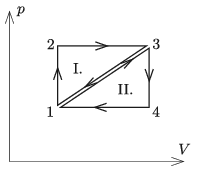(5 pont)

solution (in Hungarian), statistics

P. 5192. A mercury column confines an air column of height 40 cm and of temperature 300 K in a U-shaped tube, one end of which is closed, and which has a cross sectional area of $\displaystyle 1~\mathrm{cm}^2$, as it is shown in the figure. The external air pressure is the same as the gauge pressure of a 76 cm high mercury column.Keeping the temperature of the enclosed gas constant, some mercury of volume $\displaystyle V_0$ is poured into the tube such that the mercury levels in the two arms of the tube will be the same. Then if the enclosed air is heated we can observe that its pressure is proportional to its volume at any temperature. What is the volume $\displaystyle V_0$?

(5 pont)

solution (in Hungarian), statistics

P. 5193. Six ohmic resistors were soldered as shown in the figure. What is the equivalent resistance measured between the terminals of the $\displaystyle 20~\Omega$ resistor?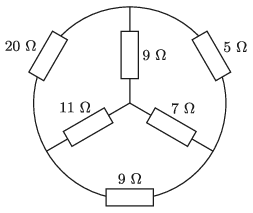(4 pont)

solution (in Hungarian), statistics

P. 5194. Consider two metal spheres of the same size, which is small compared to the 0.2 m distance between them. The two spheres are charged differently and they attract each other with a force of 1.2 N. The spheres are made to touch each other and then placed back to their original position. Now they repel each other, but the magnitude of this repulsive force is the same as the magnitude of the attractive force in the first case. What was the initial charge of the spheres?

(4 pont)

solution (in Hungarian), statistics

P. 5195. At each vertex of an equilateral triangle of sides $\displaystyle a=60$ cm there is a point-like charge of $\displaystyle Q=6\cdot 10^{-7}$ C in vacuum. What is the magnitude and the direction of the electric field at the trisection points of the sides of the triangle?

(4 pont)

solution (in Hungarian), statistics

P. 5196. A rocket engine uses the thrust obtained from the ejected exhaust. The greatest part of the energy of the propellant is the energy of the ejected exhaust, and only a small portion is converted to increase the kinetic energy of the rocket.

$\displaystyle a)$ Determine the speed increase $\displaystyle \Delta v$ of the rocket of mass $\displaystyle M$, when during some time a small amount of gas of mass $\displaystyle \Delta M$ is ejected backward at a speed of $\displaystyle u$ with respect to the rocket. (The gravitational force can be neglected in this case and in the further cases as well.)

$\displaystyle b)$ What will the speed of the rocket of initial mass $\displaystyle M_0$ be, when its mass decreases to $\displaystyle M$ $\displaystyle (M<M_0)$?

Hint: we can use that

$\displaystyle \int_{x_1}^{x_2} \frac{1}{x}\, \mathrm{d}x=\ln \frac{x_2}{x_1}.$

$\displaystyle c)$ What is the total kinetic energy of the rocket of mass $\displaystyle M$ and the ejected gas particles with respect to the reference frame of the departure?

Hint: we can use that the change in the kinetic energy of the whole system of the ejected gas and the rocket is independent of the reference frame; so it is the same in the reference frame of the moving rocket and in the reference frame of the departure.

$\displaystyle d)$ At most what can the mechanical efficiency'' of the rocket be? The mechanical efficiency is the ratio of the kinetic energy of the rocket to the total kinetic energy of the system in the reference frame of the departure.

(6 pont)

solution (in Hungarian), statistics